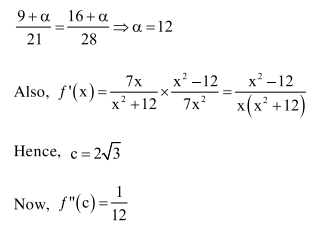# Solve this following

Question:

If $\mathrm{c}$ is a point at which Rolle's theorem holds for the function, $f(\mathrm{x})=\log _{\mathrm{e}}\left(\frac{\mathrm{x}^{2}+\alpha}{7 \mathrm{x}}\right)$ in the interval $[3,4]$, where $\alpha \in \mathrm{R}$, then $f^{\prime \prime}(\mathrm{c})$ is equal to

1. $\frac{\sqrt{3}}{7}$

2. $\frac{1}{12}$

3. $-\frac{1}{24}$

4. $-\frac{1}{12}$

Correct Option: , 2

Solution: